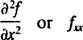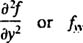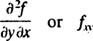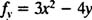## Partial Differentiation

Given a function of two variables, ƒ ( x, y), the derivative with respect to x only (treating y as a constant) is called the partial derivative of ƒ with respect to x and is denoted by either ∂ƒ / ∂ x or ƒ x. Similarly, the derivative of ƒ with respect to y only (treating x as a constant) is called the partial derivative of ƒ with respect to y and is denoted by either ∂ƒ / ∂ y or ƒ y.

The second partial dervatives of f come in four types:

Notations

• Differentiate ƒ with respect to x twice. (That is, differentiate ƒ with respect to x; then differentiate the result with respect to x again.)• Differentiate ƒ with respect to y twice. (That is, differentiate ƒ with respect to y; then differentiate the result with respect to y again.)Mixed partials:

• First differentiate ƒ with respect to x; then differentiate the result with respect to y.• First differentiate ƒ with respect to y; then differentiate the result with respect to x.For virtually all functions ƒ ( x, y) commonly encountered in practice, ƒ vx ; that is, the order in which the derivatives are taken in the mixed partials is immaterial.

Example 1: If ƒ ( x, y) = 3 x 2 y + 5 x − 2 y 2 + 1, find ƒ x , ƒ y , ƒ xx , ƒ yy , ƒ xy 1, and ƒ yx .

First, differentiating ƒ with respect to x (while treating y as a constant) yieldsNext, differentiating ƒ with respect to y (while treating x as a constant) yieldsThe second partial derivative ƒ xx means the partial derivative of ƒ x with respect to x; therefore,The second partial derivative ƒ yy means the partial derivative of ƒ y with respect to y; therefore,The mixed partial ƒ xy means the partial derivative of ƒ x with respect to y; therefore,The mixed partial ƒ yx means the partial derivative of ƒ y with respect to x; therefore,Note that ƒ yx = ƒ xy , as expected.

Back to Top
A18ACD436D5A3997E3DA2573E3FD792A